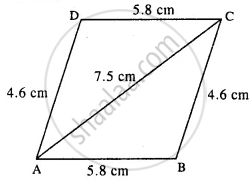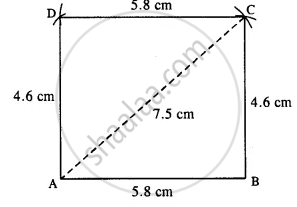# Construct a Parallelogram Abcd, If : Ab = 5.8 Cm, Ad = 4.6 Cm and Diagonal Ac = 7.5 Cm. - Mathematics

Sum

Construct a parallelogram ABCD, if :

AB = 5.8 cm, AD = 4.6 cm and diagonal AC = 7.5 cm.

#### Solution

The rough figure is as follow :opposite sides of ||gm are equal
BC = AD = 4.6 cm.
The actual figure is constructed as follow :Steps:

1. Draw AB = 5.8 cm.
2. Draw an arc of radius 4.6 cm with centre B.
3. Draw an arc of radius 7.5 cm from A which intersects the first arc at C.
4. From A, draw an arc of radius 4.6 cm.
5. From C, draw an arc of radius 5.8 cm which intersects the first arc at D.
6. Join AD, CD, BC, and AC.
Thus ABCD is the required ||gm.
Concept: Construction of Parallelograms
Is there an error in this question or solution?

#### APPEARS IN

Selina Concise Mathematics Class 8 ICSE
Chapter 18 Constructions
Exercise 18 (D) | Q 2.4 | Page 211